Showing 1 to 10 of 328 results.
0%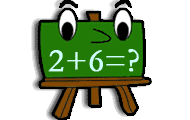• Numbers
• Mental calculation
Practice addition in math game with several levels of increasing difficulty. Add two numbers and find the correct sum.
0%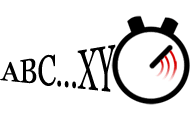## Type the alphabet

• The alphabet
• Correct fingering
• Keyboard training
Type the alphabet as fast as you can on your keyboard. Speed typing online is free to play. Type forward, backwards or in random order.
0%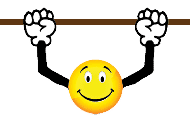## Hangman in English

• Spelling
• The alphabet
Figure out the sentence or word that is hidden by guessing letters, one at a time. Can you find the word before your hanging man falls down?
0%## Number bonds

• Numbers
• Mental calculation
• Number bonds
Try to find the number that is a number bond to another number and adds up to the given sum. Practice part-part-whole relationships to the numbers 0-20 and facilitates the important mental calculation
0%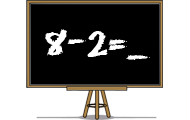## Subtraction

• Numbers
• Subtraction
• Mental calculation
Practice subtraction or minus in a skill training game. Find the difference between two numbers in different levels in this math game.
0%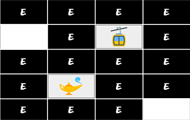## Memory

• Memory
• Strategy
Practice your brain with this memory game. There are several difficulty levels in the game, and the goal is to find and pair the images.
0%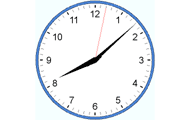## Tell time in English

• Clock
• Analog clock
• Numbers
Practice analog clock. Practice the basics such as full hours, half-hours, quarters and minutes. Watch the three clocks and choose the clock that match the requested time.
0%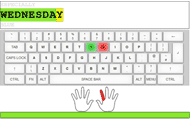## Keyboard typing

• Keyboard layout
• Touch typing
• Correct fingering
• Spelling
Practice typing on the keyboard. Being able to write correctly and quickly on the keyboard is important to use a computer to write texts. Here you can practice keyboard typing and are assisted when yo
0%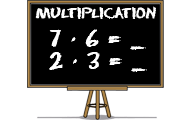## Multiplication

• Numbers
• Multiplication
• Mental calculation
Learn multiplication with this math game for students. Find the product of the factors in all times tables.
0%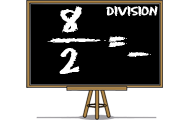## Division

• Numbers
• Division
• Mental calculation
Practice division, ie dividing two numbers. Find the quotients of the numerators divided by the denominators in this math game.
Weekly top list
1.Brain training
2.Brain training
3.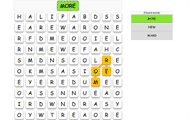English
4.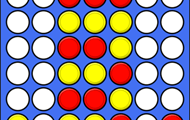Brain training
5.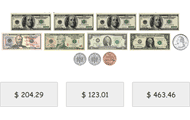Math